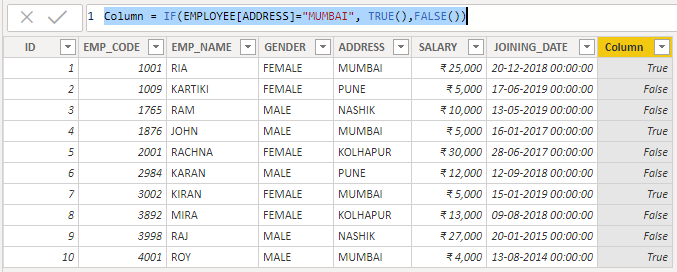# Logical DAX Function in Power BI

• Logical functions act upon an expression to return information about the values or sets in the expression.

Logical DAX Function:

• IFERROR
• TRUE
• FALSE
• SWITCH
• AND
• OR
• NOT

IF() FUNCTION:

• If the value of TRUE or FALSE is omitted, IF treats it as an empty string value (“ ”).
• Syntax: IF(logical test, Result_If_True, [Result_If_False])
• Query: Column = IF(EMPLOYEE[SALARY]<10000,” LOW SALARY “,” HIGH SALARY “)
• Output:

IFERROR() FUNCTION:

• Syntax: IFERROR(Value,Value_If_Error)
• Query: Column = IFERROR(25/0,9999)
• Output:
• Query: Column = IFERROR(BLANK(),”ERROR”)
• Output:

TRUE() FUNCTION:

• Syntax: TRUE()

FALSE() FUNCTION:

• Syntax: FALSE()
• The query of TRUE and FALSE function:

Column = IF(EMPLOYEE[ADDRESS]=”MUMBAI”, TRUE(),FALSE())RESULT OF TRUE AND FALSE STATEMENT

SWITCH() FUNCTION:

• Syntax: SWITCH()
• Query: Column = SWITCH( TRUE(),EMPLOYEE[GENDER] =

“FEMALE”, “F”, EMPLOYEE[GENDER] = “MALE”, “M” )

AND() FUNCTION:

• It accepts only two arguments. If you perform AND operation on multiple series then you can create a series of calculations or use the AND(&&) operator.
• Syntax: AND(Logical1,Logical2)
• Query: Column = IF( AND( EMPLOYEE[SALARY] < 10000, EMPLOYEE[ADDRESS] =”MUMBAI”), “MUMBAI”, “FALSE”)
• Output:

OR() FUNCTION:

• Syntax: OR(Logical1,Logical2)
• Query: Column = IF( OR ( EMPLOYEE[SALARY] > 10000, EMPLOYEE[ADDRESS] =”MUMBAI”), “TRUE”, “FALSE”)
• Output: